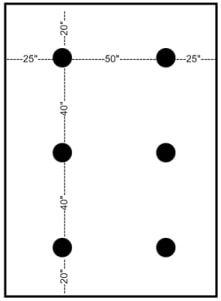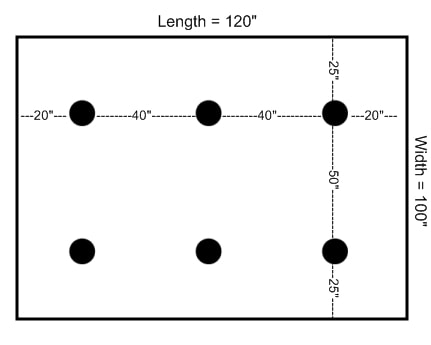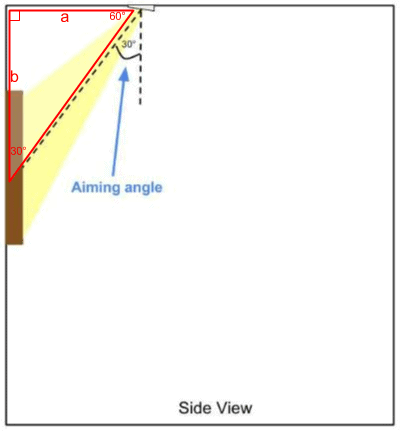# Recessed Lighting PlacementRecessed lighting placement in the ceiling is calculated based on the surface, object, or area to be illuminated.

In this post, I’ll explain how to manually calculate the placement for your lights, and then give you a calculator that does it all for you!

The formula for spacing recessed lights is the distance between the lights is always double what it is at the ends. This formula, combined with the number of lights, the layout, and the dimensions of the room or surface, is what’s used to calculate the placement of recessed lighting in the ceiling.

Note: Before you can calculate the placement, you need to know the layout for your lights.

### Calculating Placement for General and Task Lighting

1. Determine the desired “area” that is to be illuminated (It may be the whole room, a section of a larger room, or a work surface).
2. Measure the length of the area and write down your answer.
3. Divide the length of the area by twice (2x) the number of lights to be placed in that row and write down your answer. This will be the distance from the wall to your first light in that row.
4. Double (2x) your answer from the previous step and write down your answer. This will be the distance between the rest of the lights in that row. So remember, the distance between your lights is always twice (2x) the distance from the wall to the first light.[divider]
5. Now do the same for the width of the area:  Measure the width of the area and write down your answer.
6. Divide the width of the area by twice (2x) the number of lights to be placed in that row and write down your answer. This will be the distance from the wall to your first light in that row.
7. Double (2x) your answer from the previous step and write down your answer. This will be the distance between the rest of the lights in that row.

Example: Room with 6 recessed lights### Calculating Placement for Accent LightingAccent lighting is somewhat of a broad term, so I’d like to clarify that this formula is specifically for calculating the placement of recessed lights that use adjustable trims for the purpose of highlighting a painting or object on the wall.

The optimal aiming angle to minimize glare is 30-degrees from the ceiling, so that will be the starting point.

Don’t worry if the light cannot be placed at exactly 30-degrees. There may be something in the ceiling like framing or an air duct that prevents you from installing a light there. In this case you can just place the light as close to the ideal location as possible. Most accent trims have plenty of adjustment to compensate for various placements.

By using a trigonometric formula, we can calculate the ideal placement for your fixture(s).In the diagram to the right, you will notice that the accent light, the wall, and the ceiling form a right triangle. This type of triangle is known as a “30-60-90” right triangle.

Since we know all three angles of the triangle, can measure the distance of side b (from the center of your object on the wall to the ceiling), we only need to solve for a. This will be the distance away from the wall to place your accent light(s) to achieve the desired 60-degree aiming angle.

The rule for this type of triangle is that the sides always have a ratio of 1 : 2 : √3.

Using this rule and the known side of the triangle (side b), we can use the following formula to solve for side a: Side a=(Side b√3)/3.

As promised, there is a picture light calculator on this page that does it all for you!